# Tag: anabelianDavid Mumford did receive earlier this year the 2007 AMS Leroy P. Steele Prize for Mathematical Exposition. The jury honors Mumford for “his beautiful expository accounts of a host of aspects of algebraic geometry”. Not surprisingly, the first work they mention are his mimeographed notes of the first 3 chapters of a course in algebraic geometry, usually called “Mumford’s red book” because the notes were wrapped in a red cover. In 1988, the notes were reprinted by Springer-Verlag. Unfortnately, the only red they preserved was in the title.

The AMS describes the importance of the red book as follows. “This is one of the few books that attempt to convey in pictures some of the highly abstract notions that arise in the field of algebraic geometry. In his response upon receiving the prize, Mumford recalled that some of his drawings from The Red Book were included in a collection called Five Centuries of French Mathematics. This seemed fitting, he noted: “After all, it was the French who started impressionist painting and isn’t this just an impressionist scheme for rendering geometry?””

These days it is perfectly possible to get a good grasp on difficult concepts from algebraic geometry by reading blogs, watching YouTube or plugging in equations to sophisticated math-programs. In the early seventies though, if you wanted to know what Grothendieck’s scheme-revolution was all about you had no choice but to wade through the EGA’s and SGA’s and they were notorious for being extremely user-unfriendly regarding illustrations…

So the few depictions of schemes available, drawn by people sufficiently fluent in Grothendieck’s new geometric language had no less than treasure-map-cult-status and were studied in minute detail. Mumford’s red book was a gold mine for such treasure maps. Here’s my favorite one, scanned from the original mimeographed notes (it looks somewhat tidier in the Springer-version)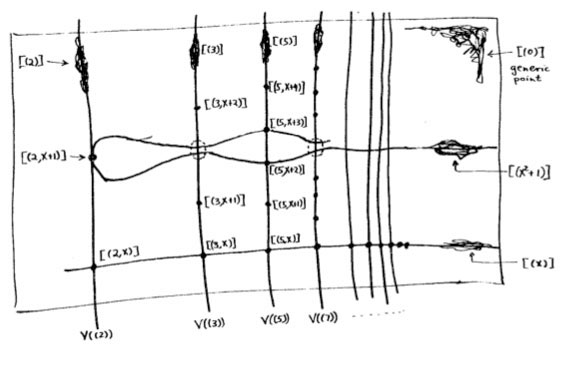It is the first depiction of $\mathbf{spec}(\mathbb{Z}[x])$, the affine scheme of the ring $\mathbb{Z}[x]$ of all integral polynomials. Mumford calls it the”arithmetic surface” as the picture resembles the one he made before of the affine scheme $\mathbf{spec}(\mathbb{C}[x,y])$ corresponding to the two-dimensional complex affine space $\mathbb{A}^2_{\mathbb{C}}$. Mumford adds that the arithmetic surface is ‘the first example which has a real mixing of arithmetic and geometric properties’.

Let’s have a closer look at the treasure map. It introduces some new signs which must have looked exotic at the time, but have since become standard tools to depict algebraic schemes.

For starters, recall that the underlying topological space of $\mathbf{spec}(\mathbb{Z}[x])$ is the set of all prime ideals of the integral polynomial ring $\mathbb{Z}[x]$, so the map tries to list them all as well as their inclusions/intersections.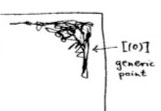The doodle in the right upper corner depicts the ‘generic point’ of the scheme. That is, the geometric object corresponding to the prime ideal $~(0)$ (note that $\mathbb{Z}[x]$ is an integral domain). Because the zero ideal is contained in any other prime ideal, the algebraic/geometric mantra (“inclusions reverse when shifting between algebra and geometry”) asserts that the gemetric object corresponding to $~(0)$ should contain all other geometric objects of the arithmetic plane, so it is just the whole plane! Clearly, it is rather senseless to depict this fact by coloring the whole plane black as then we wouldn’t be able to see the finer objects. Mumford’s solution to this is to draw a hairy ball, which in this case, is sufficiently thick to include fragments going in every possible direction. In general, one should read these doodles as saying that the geometric object represented by this doodle contains all other objects seen elsewhere in the picture if the hairy-ball-doodle includes stuff pointing in the direction of the smaller object. So, in the case of the object corresponding to $~(0)$, the doodle has pointers going everywhere, saying that the geometric object contains all other objects depicted.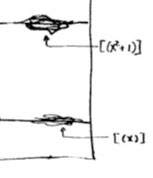Let’s move over to the doodles in the lower right-hand corner. They represent the geometric object corresponding to principal prime ideals of the form $~(p(x))$, where $p(x)$ in an irreducible polynomial over the integers, that is, a polynomial which we cannot write as the product of two smaller integral polynomials. The objects corresponding to such prime ideals should be thought of as ‘horizontal’ curves in the plane.

The doodles depicted correspond to the prime ideal $~(x)$, containing all polynomials divisible by $x$ so when we divide it out we get, as expected, a domain $\mathbb{Z}[x]/(x) \simeq \mathbb{Z}$, and the one corresponding to the ideal $~(x^2+1)$, containing all polynomials divisible by $x^2+1$, which can be proved to be a prime ideals of $\mathbb{Z}[x]$ by observing that after factoring out we get $\mathbb{Z}[x]/(x^2+1) \simeq \mathbb{Z}[i]$, the domain of all Gaussian integers $\mathbb{Z}[i]$. The corresponding doodles (the ‘generic points’ of the curvy-objects) have a predominant horizontal component as they have the express the fact that they depict horizontal curves in the plane. It is no coincidence that the doodle of $~(x^2+1)$ is somewhat bulkier than the one of $~(x)$ as the later one must only depict the fact that all points lying on the straight line to its left belong to it, whereas the former one must claim inclusion of all points lying on the ‘quadric’ it determines.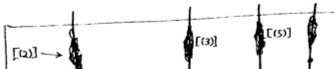Apart from these ‘horizontal’ curves, there are also ‘vertical’ lines corresponding to the principal prime ideals $~(p)$, containing the polynomials, all of which coefficients are divisible by the prime number $p$. These are indeed prime ideals of $\mathbb{Z}[x]$, because their quotients are
$\mathbb{Z}[x]/(p) \simeq (\mathbb{Z}/p\mathbb{Z})[x]$ are domains, being the ring of polynomials over the finite field $\mathbb{Z}/p\mathbb{Z} = \mathbb{F}_p$. The doodles corresponding to these prime ideals have a predominant vertical component (depicting the ‘vertical’ lines) and have a uniform thickness for all prime numbers $p$ as each of them only has to claim ownership of the points lying on the vertical line under them.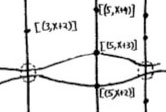Right! So far we managed to depict the zero prime ideal (the whole plane) and the principal prime ideals of $\mathbb{Z}[x]$ (the horizontal curves and the vertical lines). Remains to depict the maximal ideals. These are all known to be of the form
$\mathfrak{m} = (p,f(x))$
where $p$ is a prime number and $f(x)$ is an irreducible integral polynomial, which remains irreducible when reduced modulo $p$ (that is, if we reduce all coefficients of the integral polynomial $f(x)$ modulo $p$ we obtain an irreducible polynomial in $~\mathbb{F}_p[x]$). By the algebra/geometry mantra mentioned before, the geometric object corresponding to such a maximal ideal can be seen as the ‘intersection’ of an horizontal curve (the object corresponding to the principal prime ideal $~(f(x))$) and a vertical line (corresponding to the prime ideal $~(p)$). Because maximal ideals do not contain any other prime ideals, there is no reason to have a doodle associated to $\mathfrak{m}$ and we can just depict it by a “point” in the plane, more precisely the intersection-point of the horizontal curve with the vertical line determined by $\mathfrak{m}=(p,f(x))$. Still, Mumford’s treasure map doesn’t treat all “points” equally. For example, the point corresponding to the maximal ideal $\mathfrak{m}_1 = (3,x+2)$ is depicted by a solid dot $\mathbf{.}$, whereas the point corresponding to the maximal ideal $\mathfrak{m}_2 = (3,x^2+1)$ is represented by a fatter point $\circ$. The distinction between the two ‘points’ becomes evident when we look at the corresponding quotients (which we know have to be fields). We have

$\mathbb{Z}[x]/\mathfrak{m}_1 = \mathbb{Z}[x]/(3,x+2)=(\mathbb{Z}/3\mathbb{Z})[x]/(x+2) = \mathbb{Z}/3\mathbb{Z} = \mathbb{F}_3$ whereas $\mathbb{Z}[x]/\mathfrak{m}_2 = \mathbb{Z}[x]/(3,x^2+1) = \mathbb{Z}/3\mathbb{Z}[x]/(x^2+1) = \mathbb{F}_3[x]/(x^2+1) = \mathbb{F}_{3^2}$

because the polynomial $x^2+1$ remains irreducible over $\mathbb{F}_3$, the quotient $\mathbb{F}_3[x]/(x^2+1)$ is no longer the prime-field $\mathbb{F}_3$ but a quadratic field extension of it, that is, the finite field consisting of 9 elements $\mathbb{F}_{3^2}$. That is, we represent the ‘points’ lying on the vertical line corresponding to the principal prime ideal $~(p)$ by a solid dot . when their quotient (aka residue field is the prime field $~\mathbb{F}_p$, by a bigger point $\circ$ when its residue field is the finite field $~\mathbb{F}_{p^2}$, by an even fatter point $\bigcirc$ when its residue field is $~\mathbb{F}_{p^3}$ and so on, and on. The larger the residue field, the ‘fatter’ the corresponding point.

In fact, the ‘fat-point’ signs in Mumford’s treasure map are an attempt to depict the fact that an affine scheme contains a lot more information than just the set of all prime ideals. In fact, an affine scheme determines (and is determined by) a “functor of points”. That is, to every field (or even every commutative ring) the affine scheme assigns the set of its ‘points’ defined over that field (or ring). For example, the $~\mathbb{F}_p$-points of $\mathbf{spec}(\mathbb{Z}[x])$ are the solid . points on the vertical line $~(p)$, the $~\mathbb{F}_{p^2}$-points of $\mathbf{spec}(\mathbb{Z}[x])$ are the solid . points and the slightly bigger $\circ$ points on that vertical line, and so on.

This concludes our first attempt to decypher Mumford’s drawing, but if we delve a bit deeper, we are bound to find even more treasures… (to be continued).

In case you haven’t noticed it yet : I’m not living here anymore.

My blogging is (at least for the moment) transfered to the F_un Mathematics blog which some prefer to call the “ceci n’est pas un corps”-blog, which is very fine with me.

Javier gave a talk at MPI on Soule’s approach to algebraic geometry over the elusive field with one element $\mathbb{F}_1$ and wrote two posts about it The skeleton of Soule’s F_un geometry and Gadgets a la Soule. The rough idea being that a variety over the field with one element only acquires flesh after a base extension to $\mathbb{Z}$ and to cyclotomic integers.

I did some posts on a related (but conceptually somewhat easier) approach due to Alain Connes and Katia Consani. I’ve tried to explain their construction at the level of (mature) undergraduate students. So far, there are three posts part1, part2 and part3. Probably there is one more session to come in which I will explain why they need functors to graded sets.

In the weeks to come we plan to post about applications of this F_un-geometry to noncommutative geometry (the Bost-Connes system) and Grothendieck’s anabelian geometry (the theory of dessins d’enfant). I’ll try to leave a short account of the main posts here, but clearly you are invited to feed your feedreader this.

Perhaps I’ll return here for a week mid november to do some old-fashioned vacation blogging. I have to admit I did underestimate Numeo.fr. Rumours have it that our place is connected wirelessly to the web…

In a couple of days I’ll be blogging for 4 years… and I’m in the process of resurrecting about 300 posts from a database-dump made in june. For example here’s my first post ever which is rather naive. This conversion program may last for a couple of weeks and I apologize for all unwanted pingbacks it will produce.

I’ll try to convert chunks of related posts in one go, so that I can at least give them correct self-references. Today’s work consisted in rewriting the posts of my virtual course, in march of this year, on dessins d’enfants and its connection to noncommutative geometry (a precursor of what Ive been blogging about recently). These posts were available through the PDF-archive but are from now on open to the internal search-function. Here are the internal links and a short description of their contents

Besides, I’ve added a few scattered old posts, many more to follow…

Last time (possibly with help from the survival guide) we have seen that the universal map from the modular group $\Gamma = PSL_2(\mathbb{Z})$ to its profinite completion $\hat{\Gamma} = \underset{\leftarrow}{lim}~PSL_2(\mathbb{Z})/N$ (limit over all finite index normal subgroups $N$) gives an embedding of the sets of (continuous) simple finite dimensional representations

$\mathbf{simp}_c~\hat{\Gamma} \subset \mathbf{simp}~\Gamma$

and based on the example $\mu_{\infty} = \mathbf{simp}_c~\hat{\mathbb{Z}} \subset \mathbf{simp}~\mathbb{Z} = \mathbb{C}^{\ast}$ we would like the above embedding to be dense in some kind of noncommutative analogon of the Zariski topology on $\mathbf{simp}~\Gamma$.

We use the Zariski topology on $\mathbf{simp}~\mathbb{C} \Gamma$ as in these two M-geometry posts (( already, I regret terminology, I should have just called it noncommutative geometry )). So, what’s this idea in this special case? Let $\mathfrak{g}$ be the vectorspace with basis the conjugacy classes of elements of $\Gamma$ (that is, the space of class functions). As explained here it is a consequence of the Artin-Procesi theorem that the linear functions $\mathfrak{g}^{\ast}$ separate finite dimensional (semi)simple representations of $\Gamma$. That is we have an embedding

$\mathbf{simp}~\Gamma \subset \mathfrak{g}^{\ast}$

and we can define closed subsets of $\mathbf{simp}~\Gamma$ as subsets of simple representations on which a set of class-functions vanish. With this definition of Zariski topology it is immediately clear that the image of $\mathbf{simp}_c~\hat{\Gamma}$ is dense. For, suppose it would be contained in a proper closed subset then there would be a class-function vanishing on all simples of $\hat{\Gamma}$ so, in particular, there should be a bound on the number of simples of finite quotients $\Gamma/N$ which clearly is not the case (just look at the quotients $PSL_2(\mathbb{F}_p)$).

But then, the same holds if we replace ‘simples of $\hat{\Gamma}$’ by ‘simple components of permutation representations of $\Gamma$’. This is the importance of Farey symbols to the representation problem of the modular group. They give us a manageable subset of simples which is nevertheless dense in the whole space. To utilize this a natural idea might be to ask what such a permutation representation can see of the modular group, or in geometric terms, what the tangent space is to $\mathbf{simp}~\Gamma$ in a permutation representation (( more precisely, in the ‘cluster’ of points making up the simple components of the representation representation )). We will call this the modular content of the permutation representation and to understand it we will have to compute the tangent quiver $\vec{t}~\mathbb{C} \Gamma$.

This is how my attention was drawn to what I have since termed
anabelian algebraic geometry, whose starting point was exactly a study
(limited for the moment to characteristic zero) of the action of absolute
Galois groups (particularly the groups $Gal(\overline{K}/K)$, where K is an extension of finite type of the prime field) on (profinite) geometric fundamental
groups of algebraic varieties (defined over K), and more particularly (breaking with a well-established tradition) fundamental groups which are very far
from abelian groups (and which for this reason I call anabelian). Among
these groups, and very close to the group $\hat{\pi}_{0,3}$ , there is the profinite compactification of the modular group $SL_2(\mathbb{Z})$, whose quotient by its centre
$\{ \pm 1 \}$ contains the former as congruence subgroup mod 2, and can also be
interpreted as an oriented cartographic group, namely the one classifying triangulated oriented maps (i.e. those whose faces are all triangles or
monogons).The above text is taken from Alexander Grothendieck‘s visionary text Sketch of a Programme. He was interested in the permutation representations of the modular group $\Gamma = PSL_2(\mathbb{Z})$ as they correspond via Belyi-maps and his own notion of dessins d’enfants to smooth projective curves defined over $\overline{\mathbb{Q}}$. One can now study the action of the absolute Galois group $Gal(\overline{\mathbb{Q}}/\mathbb{Q})$ on these curves and their associated dessins. Because every permutation representation of $\Gamma$ factors over a finite quotient this gives an action of the absolute Galois group as automorphisms on the profinite compactification

$\hat{\Gamma} = \underset{\leftarrow}{lim}~\Gamma/N$

where the limit is taken over all finite index normal subgroups $N \triangleleft PSL_2(\mathbb{Z})$. In this way one realizes the absolute Galois group as a subgroup of the outer automorphism group of the profinite group $\hat{\Gamma}$. As a profinite group is a compact topological group one should study its continuous finite dimensional representations which are precisely those factoring through a finite quotient. In the case of $\hat{\Gamma}$ the simple continuous representations $\mathbf{simp}_c~\hat{\Gamma}$ are precisely the components of the permutation representations of the modular group. So in a sense, anabelian geometry is the study of these continuous simples together wirth the action of the absolute Galois group on it.

In noncommutative geometry we are interested in a related representation theoretic problem. We would love to know the simple finite dimensional representations $\mathbf{simp}~\Gamma$ of the modular group as this would give us all simples of the three string braid group $B_3$. So a natural question presents itself : how are these two ‘geometrical’ objects $\mathbf{simp}_c~\hat{\Gamma}$ (anabelian) and $\mathbf{simp}~\Gamma$ (noncommutative) related and can we use one to get information about the other?

This is all rather vague so far, so let us work out a trivial case to get some intuition. Consider the profinite completion of the infinite Abelian group

$\hat{\mathbb{Z}} = \underset{\leftarrow}{lim}~\mathbb{Z}/n\mathbb{Z} = \prod_p \hat{\mathbb{Z}}_p$

As all simple representations of an Abelian group are one-dimensional and because all continuous ones factor through a finite quotient $\mathbb{Z}/n\mathbb{Z}$ we see that in this case

$\mathbf{simp}_c~\hat{\mathbb{Z}} = \mu_{\infty}$

is the set of all roots of unity. On the other hand, the simple representations of $\mathbb{Z}$ are also one-dimensional and are determined by the image of the generator so

$\mathbf{simp}~\mathbb{Z} = \mathbb{C} – { 0 } = \mathbb{C}^*$

Clearly we have an embedding $\mu_{\infty} \subset \mathbb{C}^*$ and the roots of unity are even dense in the Zariski topology. This might look a bit strange at first because clearly all roots of unity lie on the unit circle which ‘should be’ their closure in the complex plane, but that’s because we have a real-analytic intuition. Remember that the Zariski topology of $\mathbb{C}^*$ is just the cofinite topology, so any closed set containing the infinitely many roots of unity should be the whole space!

Let me give a pedantic alternative proof of this (but one which makes it almost trivial that a similar result should be true for most profinite completions…). If $c$ is the generator of $\mathbb{Z}$ then the different conjugacy classes are precisely the singletons $c^n$. Now suppose that there is a polynomial $a_0+a_1x+\ldots+a_mx^m$ vanishing on all the continuous simples of $\hat{\mathbb{Z}}$ then this means that the dimensions of the character-spaces of all finite quotients $\mathbb{Z}/n\mathbb{Z}$ should be bounded by $m$ (for consider $x$ as the character of $c$), which is clearly absurd.

Hence, whenever we have a finitely generated group $G$ for which there is no bound on the number of irreducibles for finite quotients, then morally the continuous simple space for the profinite completion

$\mathbf{simp}_c~\hat{G} \subset \mathbf{simp}~G$

should be dense in the Zariski topology on the noncommutative space of simple finite dimensional representations of $G$. In particular, this should be the case for the modular group $PSL_2(\mathbb{Z})$.

There is just one tiny problem : unlike the case of $\mathbb{Z}$ for which this space is an ordinary (ie. commutative) affine variety $\mathbb{C}^*$, what do we mean by the “Zariski topology” on the noncommutative space $\mathbf{simp}~PSL_2(\mathbb{Z})$ ? Next time we will clarify what this might be and show that indeed in this case the subset

$\mathbf{simp}_c~\hat{\Gamma} \subset \mathbf{simp}~\Gamma$

will be a Zariski closed subset!